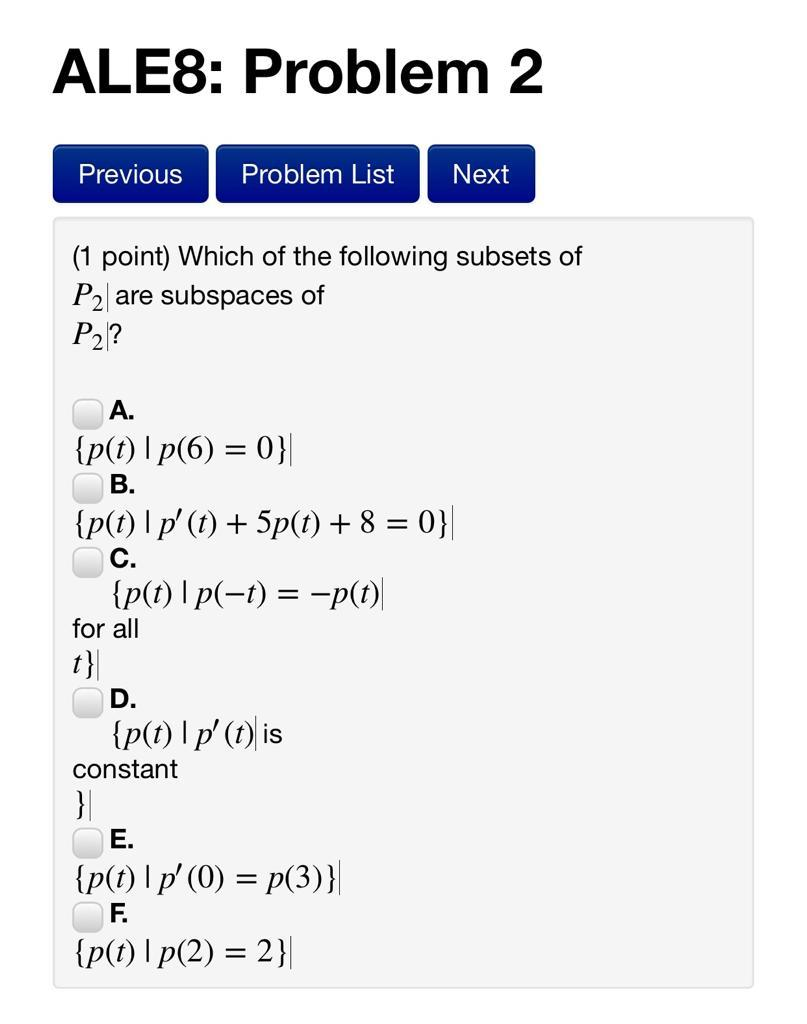### Create an Account

Already have account?

### Forgot Your Password ?

Home / Questions / ALES: Problem 2 Previous Problem List Next (1 point) Which of the following subsets of P2 ...

# ALES: Problem 2 Previous Problem List Next (1 point) Which of the following subsets of P2 are subspaces of P2)? A. {p(t) | p(6) = 0} B. {p(t)|p' (t) + 5p(t) + 8 = 0} C. {p(t) |p(-t) = -p(t) for all t}

ALES: Problem 2 Previous Problem List Next (1 point) Which of the following subsets of P2 are subspaces of P2)? A. {p(t) | p(6) = 0} B. {p(t)|p' (t) + 5p(t) + 8 = 0} C. {p(t) |p(-t) = -p(t) for all t}| D. {p(t) Ip' (t) is constant } E. {p(t)|p(0) = p(3)}| F. {p(t) | p(2) = 2}|Apr 15 2021 View more View Less

#### Answer (Solved)Subscribe To Get Solution